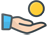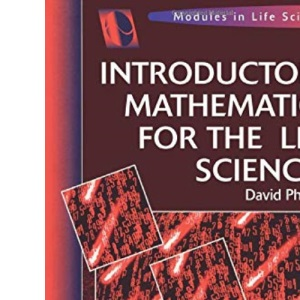30 Day Refund Guarantee# Introductory Mathematics for the Life Sciences (Lifelines)

by David Phoenix (Author)

##### Synopsis

Introductory Mathematics for the Life Sciences offers a straightforward introduction to the mathematical principles needed for studies in the life sciences. Starting with the basics of numbers, fractions, ratios, and percentages, the author explains progressively more sophisticated concepts, from algebra, measurement, and scientific notation through the linear, power, exponential, and logarithmic functions to introductory statistics. Worked examples illustrate concepts, applications, and interpretations, and exercises at the end of each chapter help readers apply and practice the skills they develop. Answers to the exercises are posted at the end of the text.

### \$3.28

Save:\$50.91 (94%)

Quantity

1 in stock